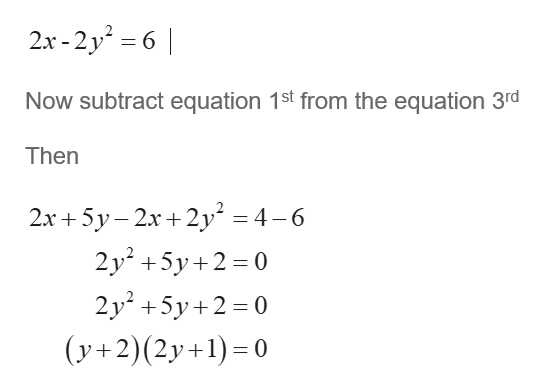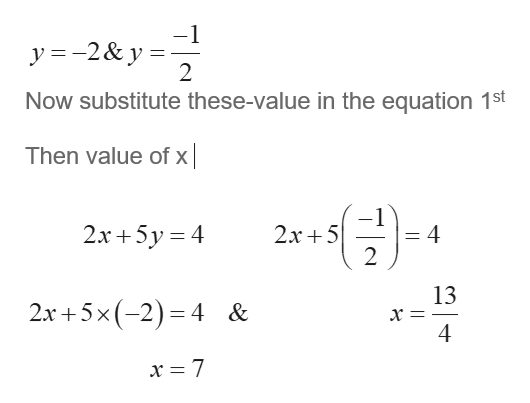# system of equations2x+5y=4x-y2 =3pairs given (6,5) (8,-4) (7,-2) and (2,6). The solution(s) of the given system of equations is/are the ordered pair(s)

Question
4 views

system of equations

2x+5y=4

x-y=3

pairs given (6,5) (8,-4) (7,-2) and (2,6).

The solution(s) of the given system of equations is/are the ordered pair(s)

check_circle

Step 1

Consider the given system of equation

Step 2

Now equation 2 multiply by 2

Thenhelp_outlineImage Transcriptionclose2x-2y- 6 Now subtract equation 1st from the equation 3rd Then 2х+5у-2х+2у* %3D 4 -6 2y2 +5y 2 0 2y +5у+2-0 (y+2)(2y+1)=0 fullscreen
Step 3

Then the value of y ...help_outlineImage Transcriptionclose-1 y 2& y 2 Now substitute these-value in the equation 1st Then value of x 2x5 2 2x+5y 4 4 = 13 х — 4 2х +5x(-2)-4 & x = 7 fullscreen

### Want to see the full answer?

See Solution

#### Want to see this answer and more?

Solutions are written by subject experts who are available 24/7. Questions are typically answered within 1 hour.*

See Solution
*Response times may vary by subject and question.
Tagged in

### Equations and In-equations